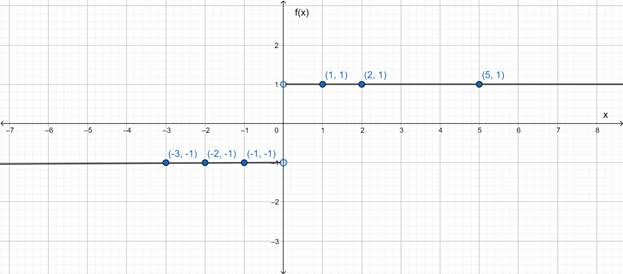# The graph of the given function.### Precalculus: Mathematics for Calcu...

6th Edition
Stewart + 5 others
Publisher: Cengage Learning
ISBN: 9780840068071### Precalculus: Mathematics for Calcu...

6th Edition
Stewart + 5 others
Publisher: Cengage Learning
ISBN: 9780840068071

#### Solutions

Chapter 2.2, Problem 28E
To determine

## To sketch : The graph of the given function.

Expert Solution

### Explanation of Solution

Given information : f(x)=x|x|

Graph :

f(x)=x|x|

 x 0 1 2 5 −1 −2 −3 f(x)=x|x| ∞ 1 1 1 −1 −1 −1The above curve is the graph of the given equation.

Interpretation : At x=0 , value of f(x) is infinite.

### Have a homework question?

Subscribe to bartleby learn! Ask subject matter experts 30 homework questions each month. Plus, you’ll have access to millions of step-by-step textbook answers!